Скачать презентацию Foreign Exchange Markets and Exchange Rates Foreign

59771e064eeaba59f0d16f5c526227a0.ppt

• Количество слайдов: 24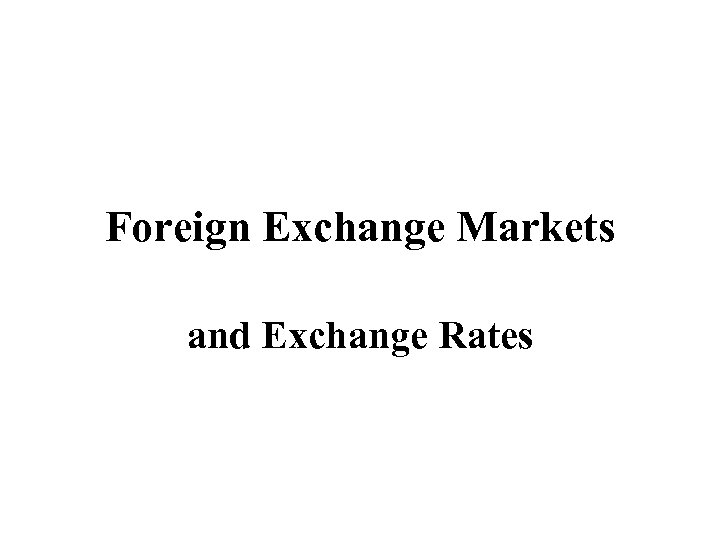Foreign Exchange Markets and Exchange Rates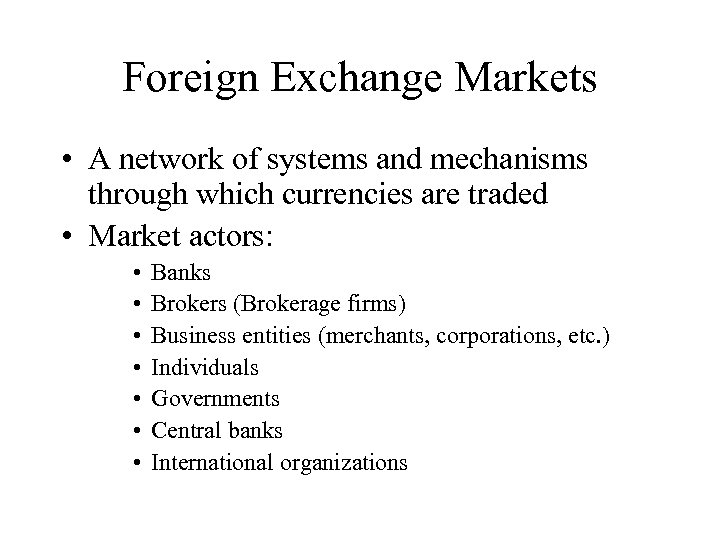Foreign Exchange Markets • A network of systems and mechanisms through which currencies are traded • Market actors: • • Banks Brokers (Brokerage firms) Business entities (merchants, corporations, etc. ) Individuals Governments Central banks International organizations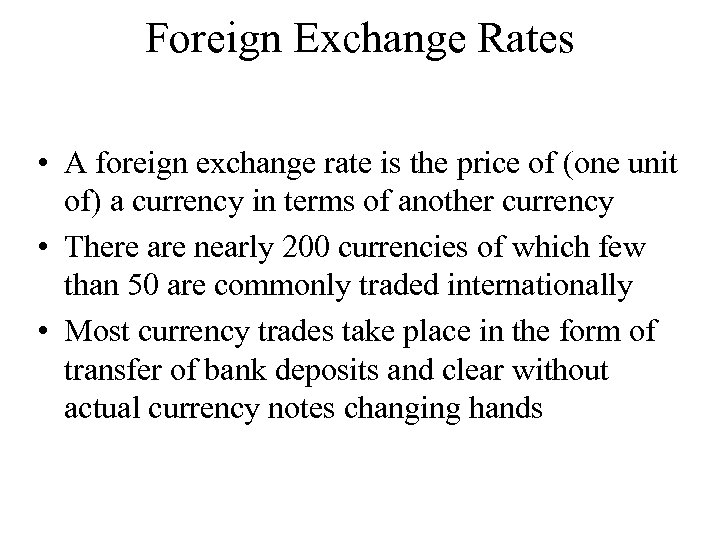Foreign Exchange Rates • A foreign exchange rate is the price of (one unit of) a currency in terms of another currency • There are nearly 200 currencies of which few than 50 are commonly traded internationally • Most currency trades take place in the form of transfer of bank deposits and clear without actual currency notes changing hands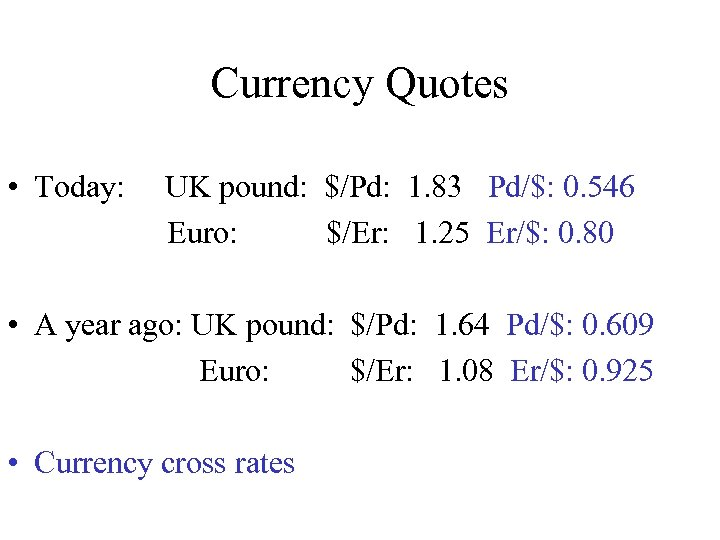Currency Quotes • Today: UK pound: \$/Pd: 1. 83 Pd/\$: 0. 546 Euro: \$/Er: 1. 25 Er/\$: 0. 80 • A year ago: UK pound: \$/Pd: 1. 64 Pd/\$: 0. 609 Euro: \$/Er: 1. 08 Er/\$: 0. 925 • Currency cross rates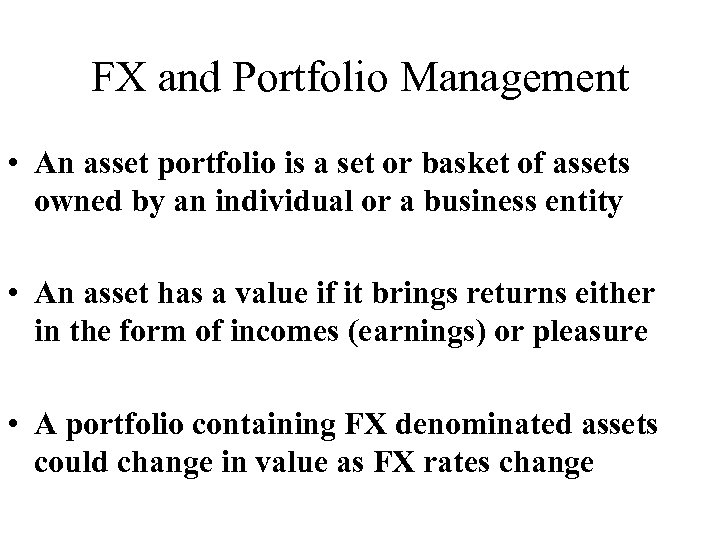FX and Portfolio Management • An asset portfolio is a set or basket of assets owned by an individual or a business entity • An asset has a value if it brings returns either in the form of incomes (earnings) or pleasure • A portfolio containing FX denominated assets could change in value as FX rates change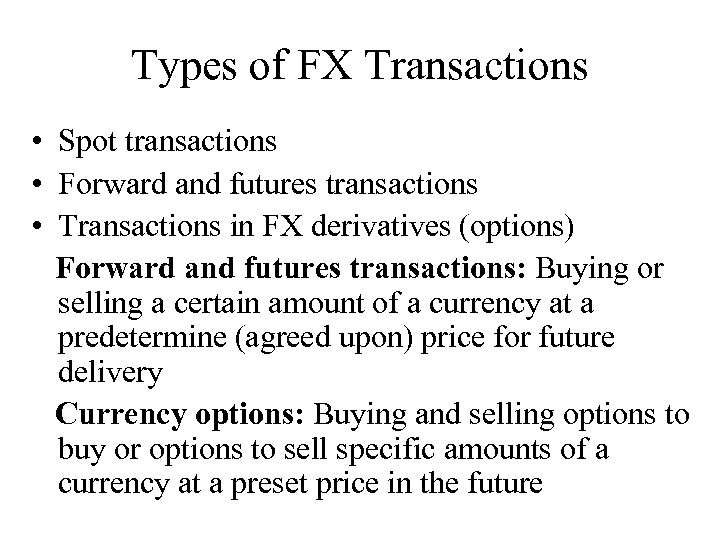Types of FX Transactions • Spot transactions • Forward and futures transactions • Transactions in FX derivatives (options) Forward and futures transactions: Buying or selling a certain amount of a currency at a predetermine (agreed upon) price for future delivery Currency options: Buying and selling options to buy or options to sell specific amounts of a currency at a preset price in the future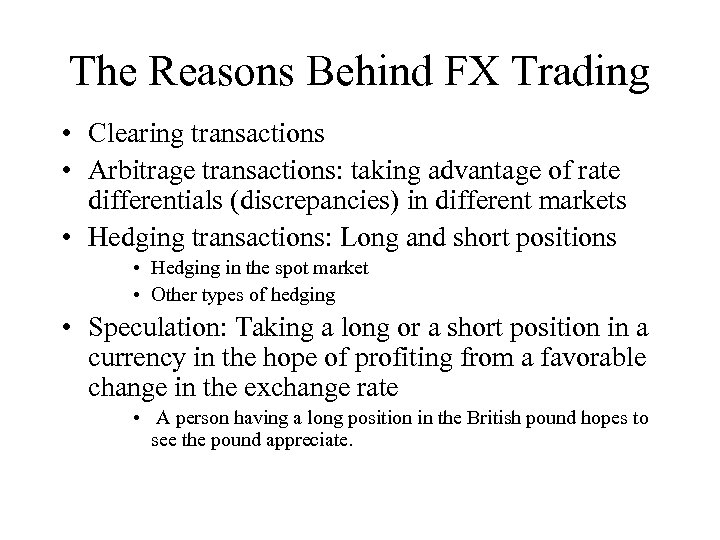The Reasons Behind FX Trading • Clearing transactions • Arbitrage transactions: taking advantage of rate differentials (discrepancies) in different markets • Hedging transactions: Long and short positions • Hedging in the spot market • Other types of hedging • Speculation: Taking a long or a short position in a currency in the hope of profiting from a favorable change in the exchange rate • A person having a long position in the British pound hopes to see the pound appreciate.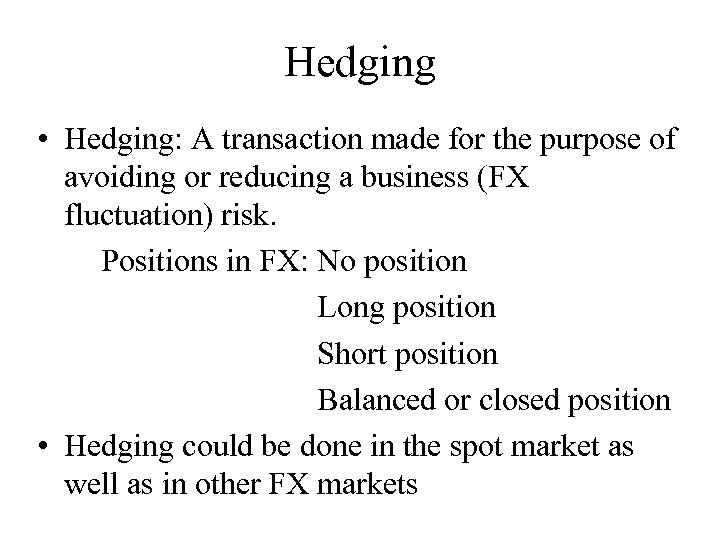Hedging • Hedging: A transaction made for the purpose of avoiding or reducing a business (FX fluctuation) risk. Positions in FX: No position Long position Short position Balanced or closed position • Hedging could be done in the spot market as well as in other FX markets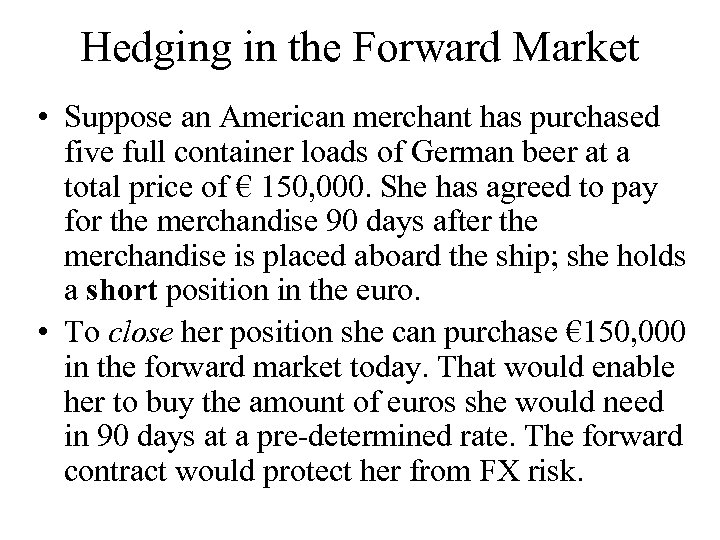Hedging in the Forward Market • Suppose an American merchant has purchased five full container loads of German beer at a total price of € 150, 000. She has agreed to pay for the merchandise 90 days after the merchandise is placed aboard the ship; she holds a short position in the euro. • To close her position she can purchase € 150, 000 in the forward market today. That would enable her to buy the amount of euros she would need in 90 days at a pre-determined rate. The forward contract would protect her from FX risk.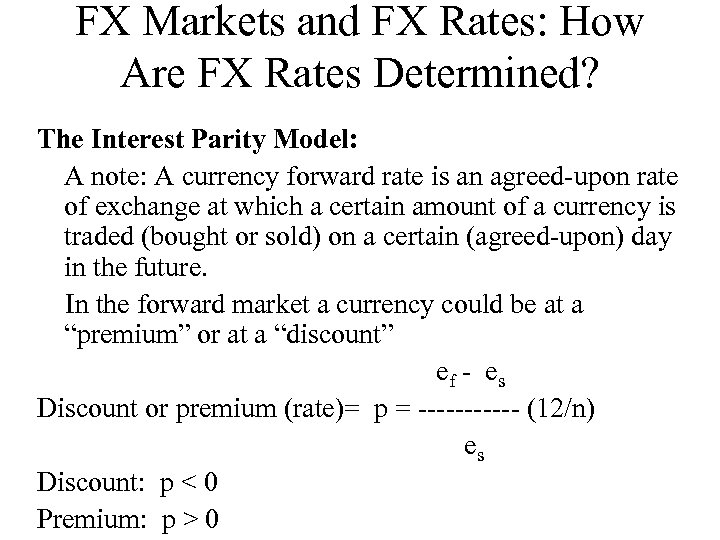FX Markets and FX Rates: How Are FX Rates Determined? The Interest Parity Model: A note: A currency forward rate is an agreed-upon rate of exchange at which a certain amount of a currency is traded (bought or sold) on a certain (agreed-upon) day in the future. In the forward market a currency could be at a “premium” or at a “discount” ef - es Discount or premium (rate)= p = ------ (12/n) es Discount: p < 0 Premium: p > 0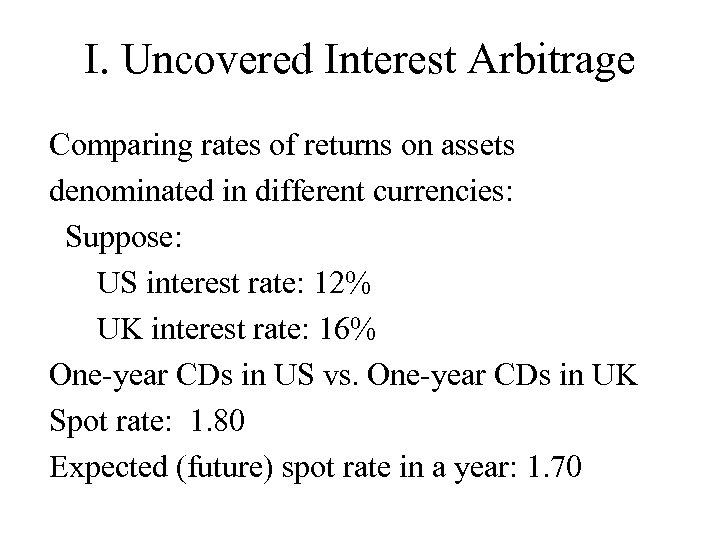I. Uncovered Interest Arbitrage Comparing rates of returns on assets denominated in different currencies: Suppose: US interest rate: 12% UK interest rate: 16% One-year CDs in US vs. One-year CDs in UK Spot rate: 1. 80 Expected (future) spot rate in a year: 1. 70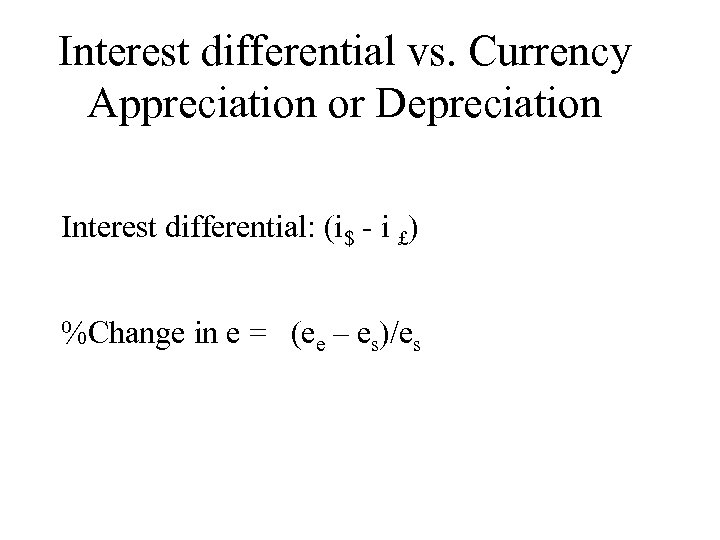Interest differential vs. Currency Appreciation or Depreciation Interest differential: (i\$ - i £) %Change in e = (ee – es)/es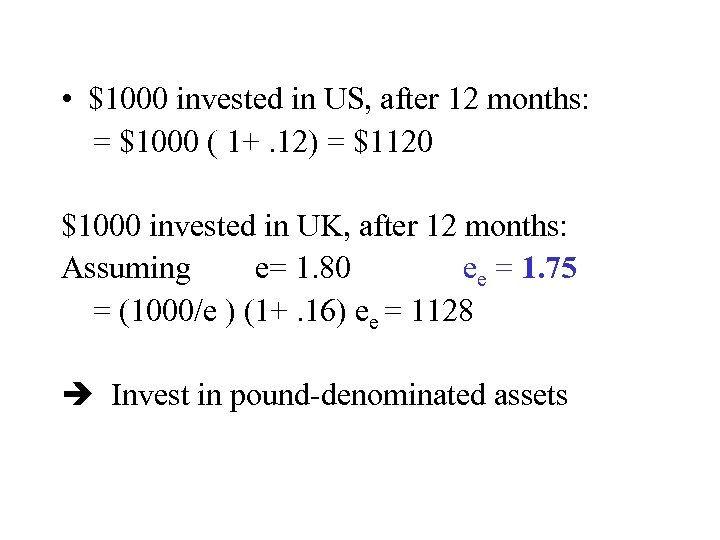• \$1000 invested in US, after 12 months: = \$1000 ( 1+. 12) = \$1120 \$1000 invested in UK, after 12 months: Assuming e= 1. 80 ee = 1. 75 = (1000/e ) (1+. 16) ee = 1128 Invest in pound-denominated assets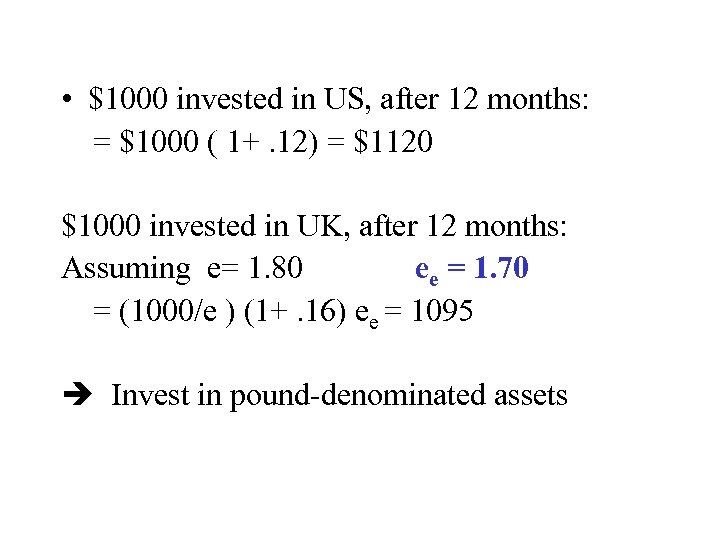• \$1000 invested in US, after 12 months: = \$1000 ( 1+. 12) = \$1120 \$1000 invested in UK, after 12 months: Assuming e= 1. 80 ee = 1. 70 = (1000/e ) (1+. 16) ee = 1095 Invest in pound-denominated assets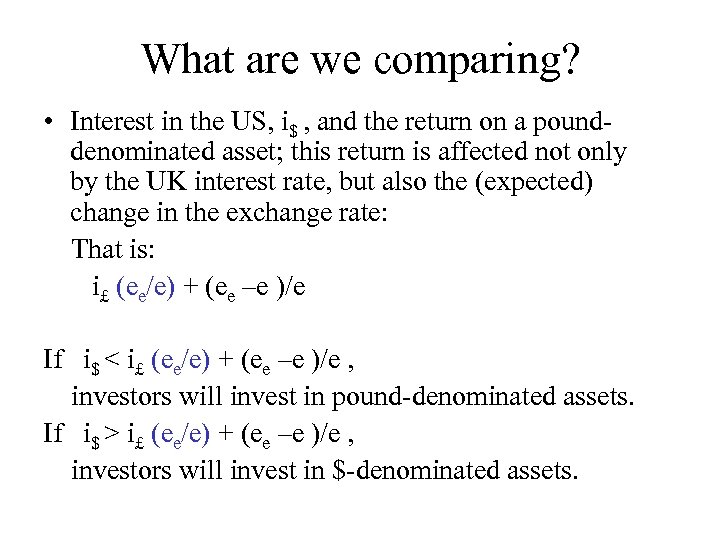What are we comparing? • Interest in the US, i\$ , and the return on a pounddenominated asset; this return is affected not only by the UK interest rate, but also the (expected) change in the exchange rate: That is: i£ (ee/e) + (ee –e )/e If i\$ < i£ (ee/e) + (ee –e )/e , investors will invest in pound-denominated assets. If i\$ > i£ (ee/e) + (ee –e )/e , investors will invest in \$-denominated assets.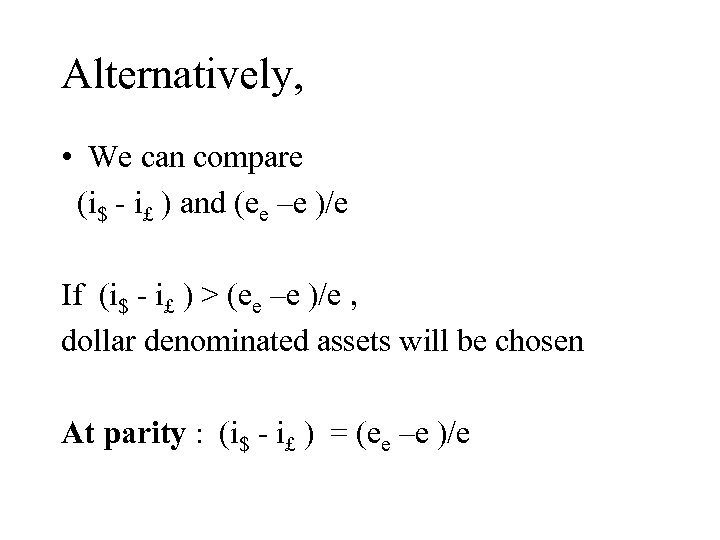Alternatively, • We can compare (i\$ - i£ ) and (ee –e )/e If (i\$ - i£ ) > (ee –e )/e , dollar denominated assets will be chosen At parity : (i\$ - i£ ) = (ee –e )/e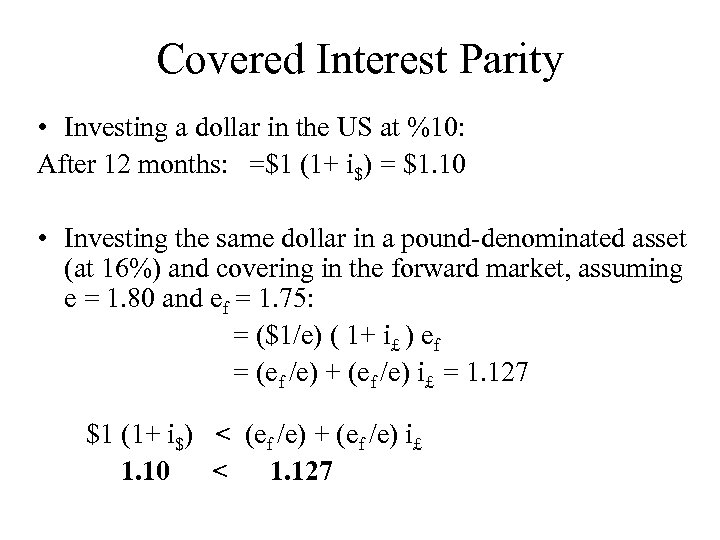Covered Interest Parity • Investing a dollar in the US at %10: After 12 months: =\$1 (1+ i\$) = \$1. 10 • Investing the same dollar in a pound-denominated asset (at 16%) and covering in the forward market, assuming e = 1. 80 and ef = 1. 75: = (\$1/e) ( 1+ i£ ) ef = (ef /e) + (ef /e) i£ = 1. 127 \$1 (1+ i\$) < (ef /e) + (ef /e) i£ 1. 10 < 1. 127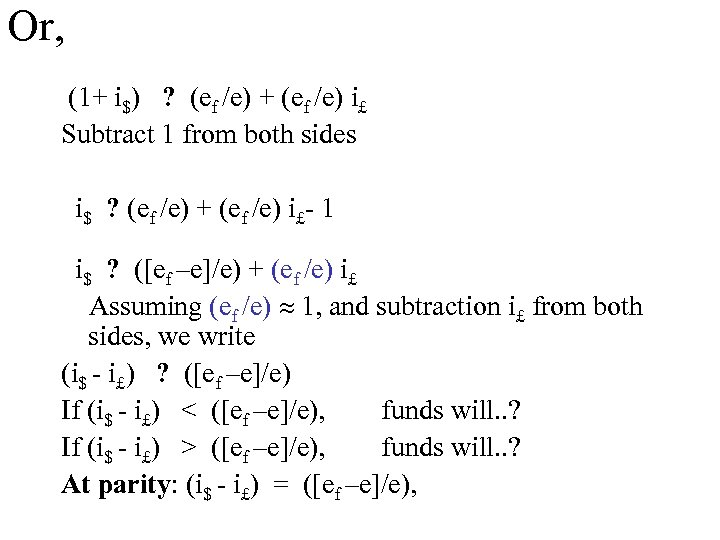Or, (1+ i\$) ? (ef /e) + (ef /e) i£ Subtract 1 from both sides i\$ ? (ef /e) + (ef /e) i£- 1 i\$ ? ([ef –e]/e) + (ef /e) i£ Assuming (ef /e) 1, and subtraction i£ from both sides, we write (i\$ - i£) ? ([ef –e]/e) If (i\$ - i£) < ([ef –e]/e), funds will. . ? If (i\$ - i£) > ([ef –e]/e), funds will. . ? At parity: (i\$ - i£) = ([ef –e]/e),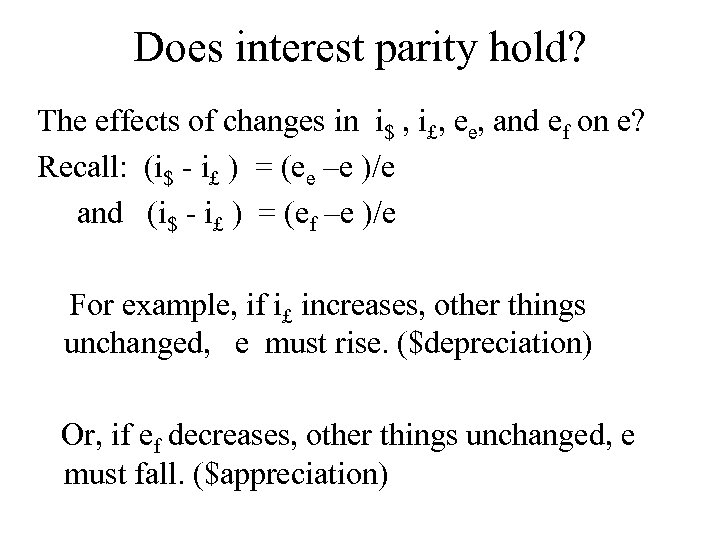Does interest parity hold? The effects of changes in i\$ , i£, ee, and ef on e? Recall: (i\$ - i£ ) = (ee –e )/e and (i\$ - i£ ) = (ef –e )/e For example, if i£ increases, other things unchanged, e must rise. (\$depreciation) Or, if ef decreases, other things unchanged, e must fall. (\$appreciation)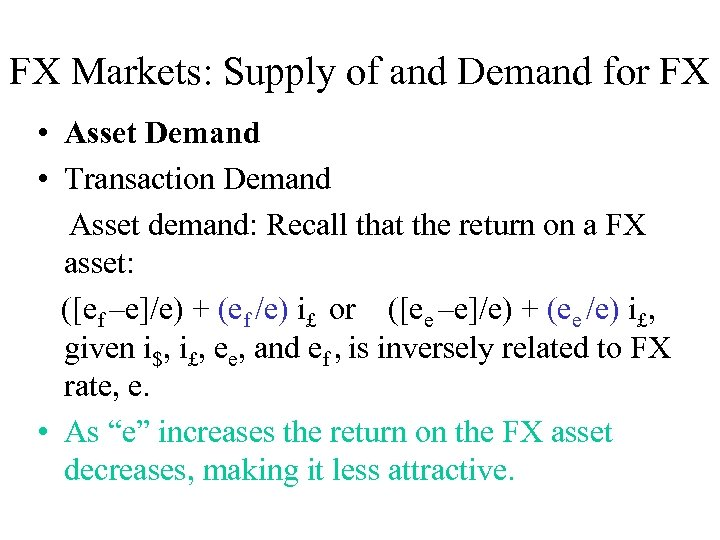FX Markets: Supply of and Demand for FX • Asset Demand • Transaction Demand Asset demand: Recall that the return on a FX asset: ([ef –e]/e) + (ef /e) i£ or ([ee –e]/e) + (ee /e) i£, given i\$, i£, ee, and ef , is inversely related to FX rate, e. • As “e” increases the return on the FX asset decreases, making it less attractive.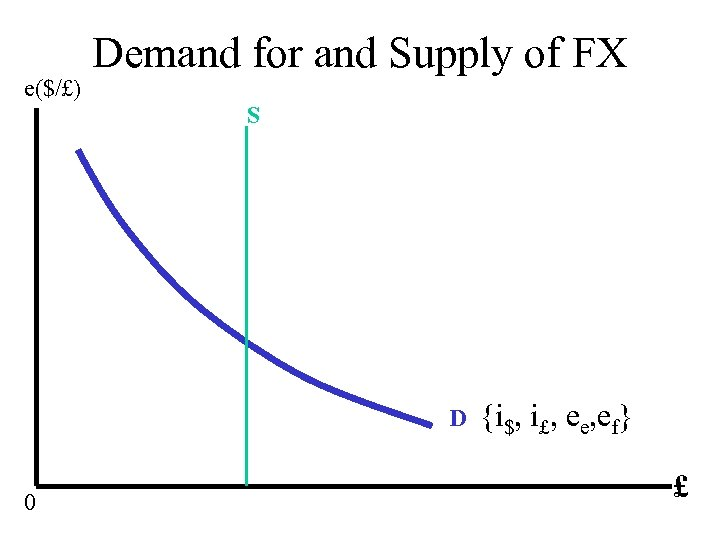e(\$/£) Demand for and Supply of FX S D 0 {i\$, i£, ee, ef} £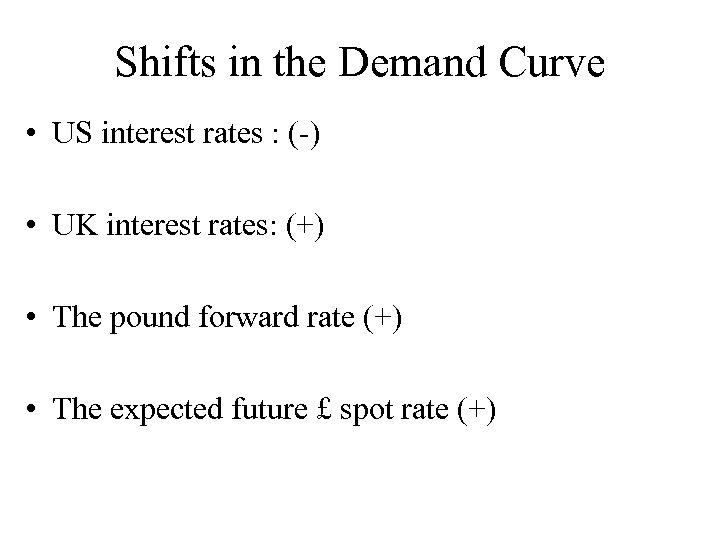Shifts in the Demand Curve • US interest rates : (-) • UK interest rates: (+) • The pound forward rate (+) • The expected future £ spot rate (+)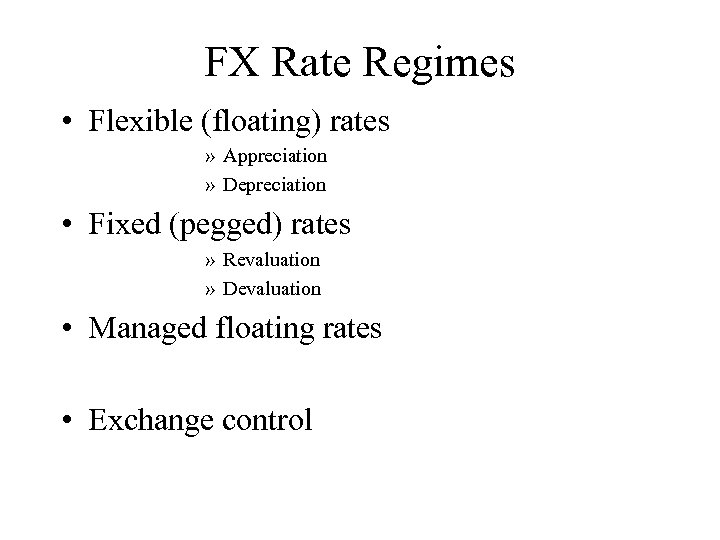FX Rate Regimes • Flexible (floating) rates » Appreciation » Depreciation • Fixed (pegged) rates » Revaluation » Devaluation • Managed floating rates • Exchange control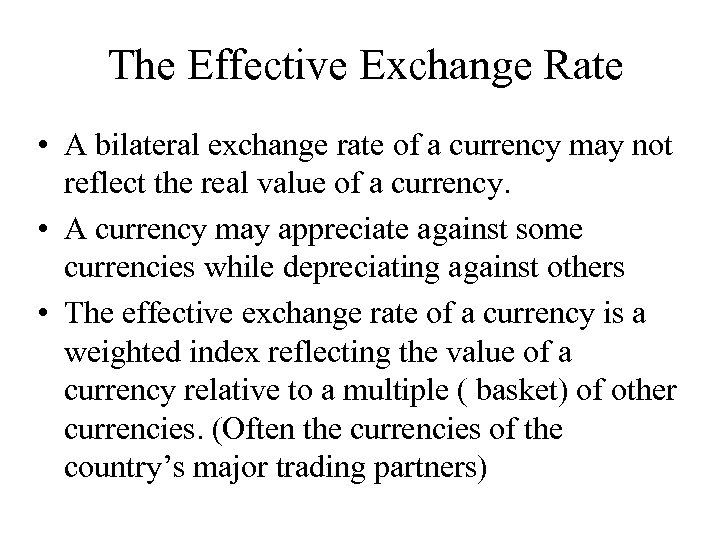The Effective Exchange Rate • A bilateral exchange rate of a currency may not reflect the real value of a currency. • A currency may appreciate against some currencies while depreciating against others • The effective exchange rate of a currency is a weighted index reflecting the value of a currency relative to a multiple ( basket) of other currencies. (Often the currencies of the country’s major trading partners)